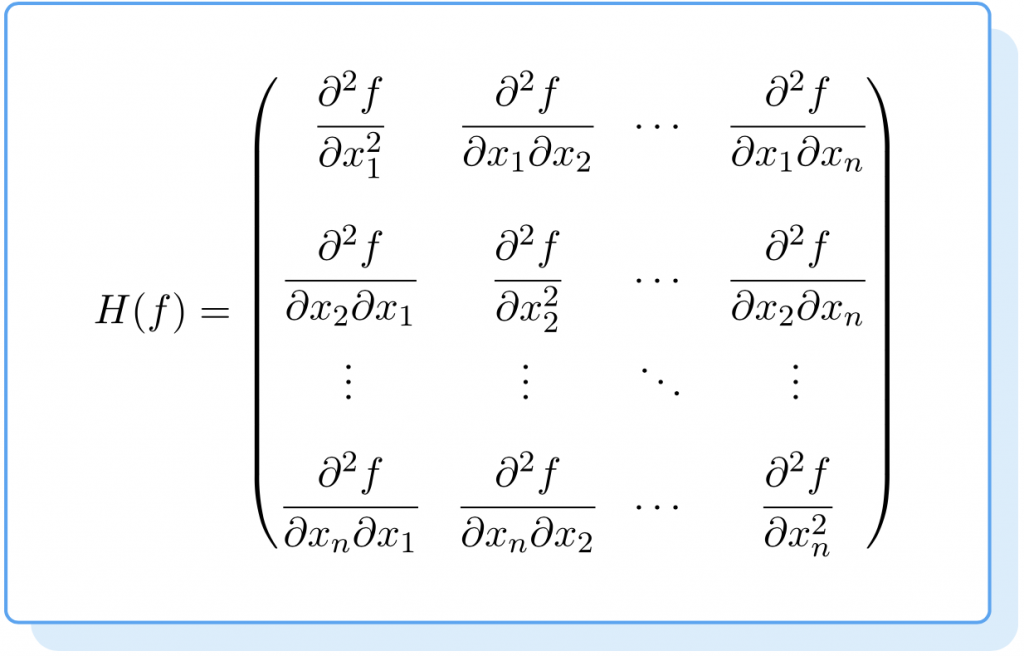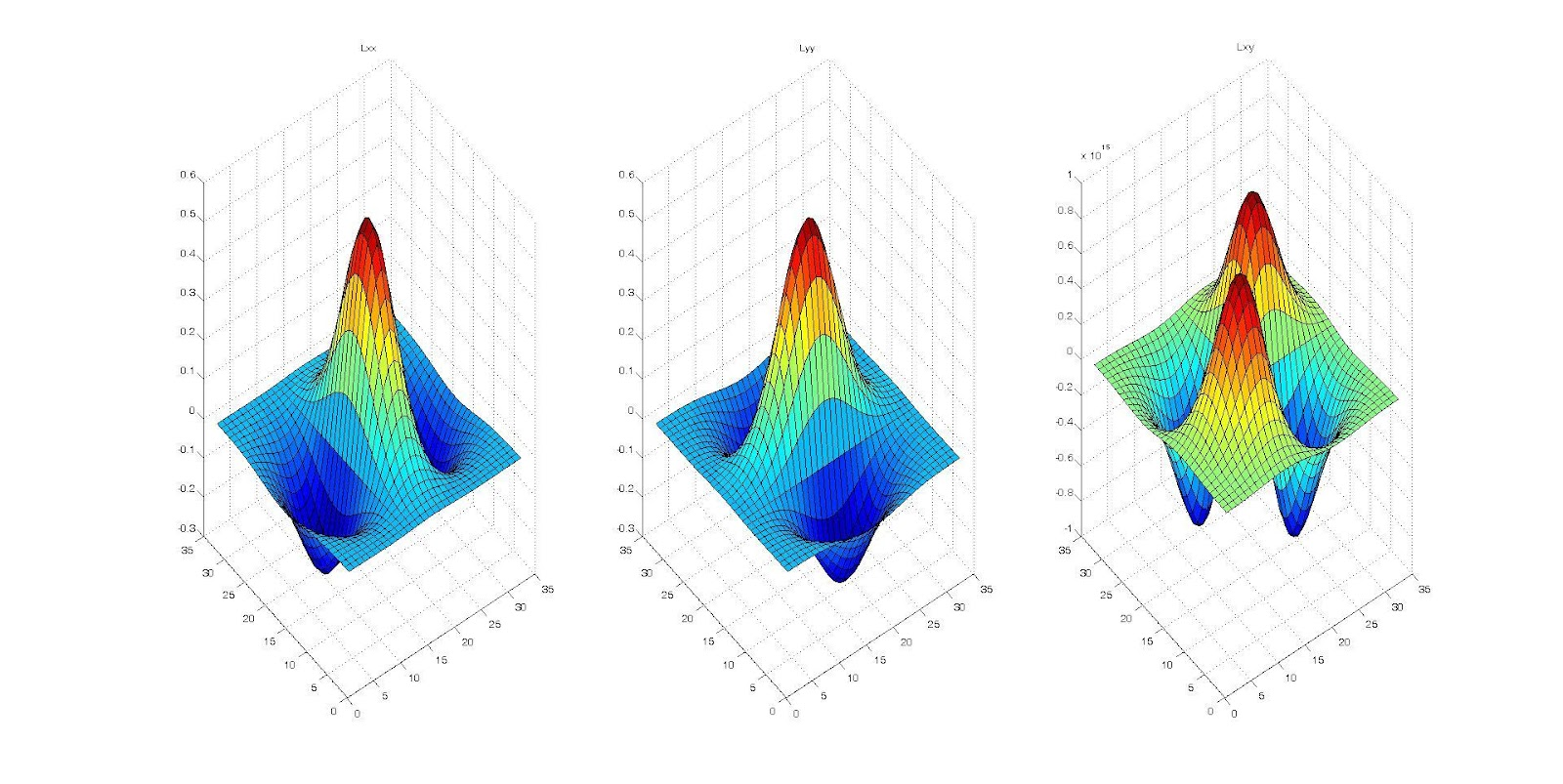##### Hessian matrix

Hessian matrices are square matrices of second-order partial derivatives of functions or fields with scalar values. With many variables, it describes the local curvature of the function.

Nov. 27, 2022 1 minuteHessian matrices are square matrices of second-order partial derivatives of functions or fields with scalar values. With many variables, it describes the local curvature of the function.

Ludwig Otto Hesse developed the Hessian matrix in the 19th century and named it after him later. According to Hesse, functional determinants were originally called "functional determinants".

## What is a hessian matrix?

Hessian matrices are square matrices of second-order partial derivatives. This function is extremely useful in linear algebra and determining local maxima or minima.

## How to calculate the hessian matrix?

Hessian matrices have the following formula:In other words, the Hessian matrix will always be a square matrix whose dimension equals the number of variables in the function. As an example, if the function has three variables, the Hessian matrix will be a 3x3 matrix.

## How do we find the eigenvalues of a hessian matrix?

Eigenvalues are data points that describe a matrix. Hessian matrices contain geometric information about surfaces z=f(x,y). Geometric information can be obtained from the eigenvalues of the Hessian matrix.## Conclusion

In mathematics, a Hessian matrix is a square matrix of second-order partial derivatives of a scalar function or field used to determine local maxima or minima. A Hessian matrix is always a square matrix whose dimension equals the number of variables in the function. To obtain geometric information, we will use the eigenvalues of the Hessian matrix.